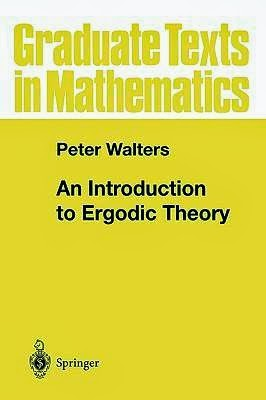## Thursday, March 27, 2014

### Walters: An Introduction to Ergodic Theory (1982), p. 26Book cover; from Booktopia.
Section 1.4 of Peter Walters' textbook on ergodic theory contains a proof of Poincaré's recurrence theorem. I found it a little difficult to read, so I'll try to paraphrase the proof here using a vocabulary that might be a bit more intuitive.

### The Possible is the Necessarily Possible

The theorem states the following: If
1. X is a probability space
2. T: XX is a measure-preserving transformation of X,
3. E is an event with positive probability,
4. and x a point in E,
then the series
x, Tx, T2x, T3x, T4x, …
will almost certainly pass through E infinitely often. Or: if it happens once, it will happen again.

The idea behind the proof is to describe the set R of points that visit E infinitely often as the superior limit of a series of sets. This description can then be used to show that ER has the same measure as E. This will imply that almost all points in E revisit E infinitely often.Statement and proof of the theorem; scan from page 26 in Walters' book.

I'll try to spell this proof out in more detail below. My proof is much, much longer than Walters', but hopefully this means that it's much, much more readable.

### Late Visitors

Let Ri be the set of points in X that visit E after i or more applications of T. We can then make two observations about the series R0, R1, R2, R3, …:

First, if j > i, and you visit E at a time later than j, you also visit E at a time later than i. The Ri's are consequently nested inside each other:
R0R1R2R3 ⊃ …
Let's use the name R for the limit of this series (that is, the intersection of the sets). R then consists of all the points in X that visit E infinitely often.The series of sets is downward converging.

Second, Ri contains the points that visit E at time i or later, and the transformation T–1 takes us one step back in time. The set T–1Ri consequently contains the points in X that visit E at time i + 1 or later. Thus
T–1Ri = Ri + 1.
But since we have assumed that T is measure-preserving, this implies that
m(Ri) = m(T–1Ri) = m(Ri + 1).
By induction, every set in the series thus has the same measure:
m(R0) = m(R1) = m(R2) = m(R3) = …
Or to put is differently, the discarded parts R0\R1, R1\R2, R2\R3, etc., are all null sets.

### Intersection by Intersection

So we have that
1. in set-theoretic terms, the Ri's converge to a limit R from above;
2. but all the Ri's have the same measure.
Let's use these facts to show that m(ER) = m(E), that is, that we only throw away a null set by intersecting E with R.The event E and the set R of points that visit the E infinitely often.

To prove this, notice first that every point in E visits E after zero applications of T. Thus, R0E, or in other words, ER0 = E. Consequently,
m(ER0) = m(E).
We now need to extend this base case by a limit argument to show that
m(ER) = m(E).
But, as we have seen above, the difference between R0 and R1 is a null set. Hence, the difference between ER0 and ER1 is also a null set, so
m(ER0) = m(ER1).
This argument holds for any i and i + 1. By induction, we thus get
m(ER0) = m(ER1) = m(ER2) = m(ER3) = …The probability of a visit to E before but never after time i has probability 0.

Since measures respect limits, this implies that
m(ER0) = m(ER).
But we have already seen that m(ER0) = m(E), this implies that
m(ER) = m(E).

### The Wherefore

An informal explanation of what's going on in this proof might be the following:

We are interested in the conditional probability of visiting E infinitely often given that we have visited it once, that is, Pr(R | E). In order to compute this probability, we divide up RE into an infinite number of cases and discover that all but one of them have probability 0.

If you Imagine yourself walking along a sample path, your route will fall in one of the following categories:
• you never visit E;
• you visit E for the last time at time i = 0;
• you visit E for the last time at time i = 1;
• you visit E for the last time at time i = 2;
• you visit E for the last time at time i = 3;
• there is no last time — i.e., you visit E infinitely often.
When we condition on E, the first of these cases has probability 0.

In general, the fact that T is measure-preserving guarantees that it is impossible for an event to occur i times without occurring i + 1 times; consequently, all of the following cases also have probability 0.

We thus have to conclude that the last option — infinitely many visits to E — has the same probability as visiting E once, and thus a conditional probability of 1.